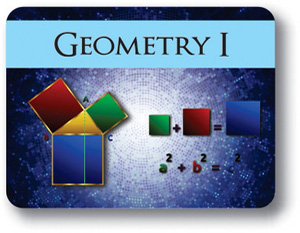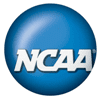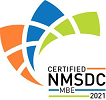Red Comet## Geometry - Semester - 1

#### Course Price: \$285.00## Course Details:

This course provides an opportunity for students to explore geometric relationships. Students perform constructions, measure figures, observe patterns, discuss their findings, write their own definitions, and formulate and prove geometric conjectures. Topics include methods of reasoning; relations in Geometry; properties of lines, circles and angle, geometric transformations; similarities, applications and properties of triangles; transformations of the Euclidean plane and several concepts applicable in quadrilaterals.

Note: This course is not designed for ELL (English Language Learners) students. ELL students may enroll in this course ONLY if they have adequate mentor support at their home school and are able to fulfill all course requirements.

## Syllabus:

Section 1 - Introduction to Geometry

Objectives:

• Find the difference between Deductive and Inductive Method of Reasoning
• The importance of parallel postulate, and how parallel postulate affect the Euclidean and Non-Euclidean geometry.
• Master Basic Concepts and Basic Relations in Euclidean Geometry
• Demonstrate an ability to prove geometric theorems using axioms, basics terms and previously proved theorems.
• Identify parallel, perpendicular and skew lines.

Lessons:

• Deductive and Inductive Method of Reasoning
• A Brief History of Geometry
• Basic Concepts and Basic Relations in Geometry
• Midpoint, Angle and Circle
• Parallel and Perpendicular lines
• Measuring Angles
• Angle Pair Relations and Angle Bisector
• Basic Constructions with a Variety Tools and Methods

Section 2 - Angles And Triangles - Transformation and Congruence

Objectives:

• Recognize and identify a transversal of two lines and corresponding angle pairs
• Find angle pair measurements of angles on transversal
• Differences between Rigid and Non-Rigid Transformations
• Identify congruent triangles in terms of rigid motion
• Identify the corresponding parts of congruent triangles

Lessons:

• Angles on Transversal
• Introduction to Triangles
• Function Transformation
• Geometric Transformations
• Rigid and Non-Rigid Transformations
• Congruence of Triangles
• Congruence-Isosceles, Equilateral, and Right Triangles
• Basic Constructions
• Constructions of Triangles

Section 3 - Transformations of the Euclidean Plane

Objectives:

• Describe the result of a rigid motion on two-dimensional figures
• Identify basic rigid transformation rotation, translation, reflection and dilatation
• Predict the result of transformations
• Visualize transformations in the two-dimensional Cartesian plane
• Identify if a figure has reflectional symmetry or rotational symmetry. Find the lines of symmetry and points of symmetry.

Lessons:

• Reflection
• Translation
• Rotation
• Dilation
• Interactive Geometry Software-GeoGebra

Section 4 - Inequalities in Triangles & Concepts in Quadrilaterals

Objectives:

• How to use inequalities in a triangle involving angles
• How to use inequalities in a triangle involving sides
• Find if it’s possible to construct a triangle with three given line segments
• Sort and classify quadrilaterals
• Find the differences between quadrilaterals
• Discover the properties and applications of a special type of quadrilaterals

Lessons:

• Inequalities in Triangle
• Basic Constructions of Quadrilaterals
• Rhombi, Rectangles and Squares
• Midsegments of Triangle and Trapezoid

Section 5 - Triangles - Similarity and Applications

Objectives:

• Solve different problems using ratio and proportion
• Explore some important aspects of triangles such as centroid, orthocenter, circumcenter and incenter
• Explore some important segments in triangles such as altitudes, medians, circumradius, inradius
• Study similar polygons and triangles
• Determine and use relations in similar triangles to find a missing measure
• Using triangle similarity to solve different problems
• Apply the similarity and similarity properties in right triangles

Lessons:

• Some Important Points of Triangles
• Ratio and Proportion
• Similarity of Figures
• Criteria for Similarity of Triangles
• Further Application of Similarity
• Constructions Using Similarity

### Accreditation & Approvals